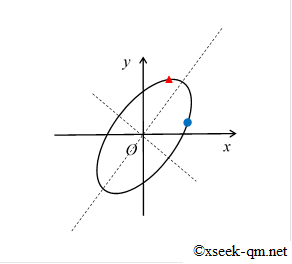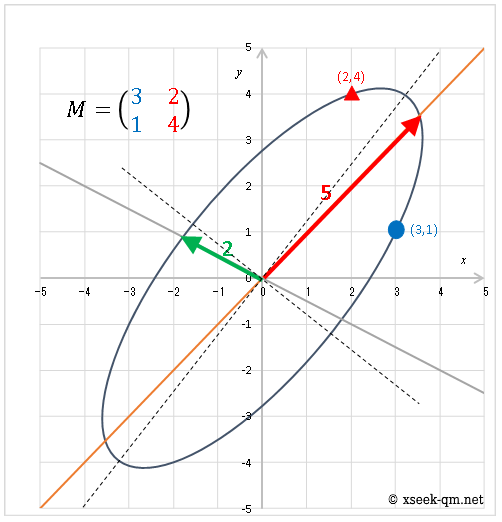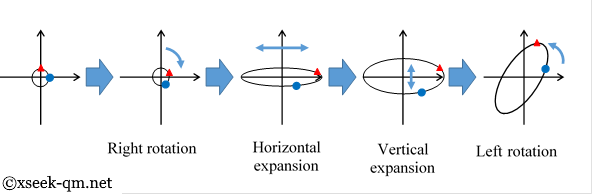# Do imaginary numbers exist?

2015/7/5
Published 2014/6/29

Imaginary numbers are transformations to expand and rotate an image 180 degrees by twice transforming.Imaginary unit matrix

In the next article, we explain matrix representations of imaginary numbers.

## Do imaginary numbers exist?

I worried very much, when I learned imaginary numbers for the first time.The real numbers which become -1 do not exist when they are squared. So I felt big uneasiness to suppose the existence of imaginary numbers. If we defined such strange numbers, I thought that contradiction might occur somewhere. However, the contradiction does not appear at all everywhere. It goes well, like a puzzle which the necessary pieces are fitting into. Therefore, I began to have a different question.

"Why it works, though we suppose the impossible number?"

## Matrix representations of imaginary numbers

Although I was continuing worrying with such a question, I met with a certain problem of mathematics one day. The problem defined the following matrix.This matrix I is called the matrix representation of an imaginary number. The operation of this matrix becomes just same as an operation of an imaginary number. For example, we have the following equation.After I met this problem, I interpreted as follows.

"A symbol i of an imaginary number is an abbreviation of a matrix I".

I explain the meaning of the sentence, "an imaginary number is an abbreviation of a matrix". For example, we assume that we met the following quadratic equation.We interpret this equation as the following equation of a matrix X.Then, we naturally obtain the following solution.We interpret this solution as the following solution.My question was cleared by interpreting it like that. I was able to be convinced.

### The explanation of complex numbers by matrices

I think that it is better not to explain complex numbers in the view point of quadratic equations. We can't help but have the impression that the imaginary numbers are numbers which do not exist, if we see imaginary numbers in the view point of quadratic equations. I think that we had better explain the imaginary numbers in the view point of the matrices. It is a totally impossible phenomenon that the square of the number becomes -1, if we see the imaginary numbers in the view point of quadratic equations. On the other hand, It is a phenomenon possible enough that the square of the matrix becomes minus unit matrix –E, if we see the imaginary numbers in the view point of the matrices.

So, I made the following article to guide the complex from the matrix. In the article, I call the above matrix I imaginary unit matrix or rotation unit matrix.

The purpose of the article is to reduce the person who suffers from imaginary numbers like me. The article needs the knowledge of the matrices. Therefore the target reader of the article will be the person who learned matrices.

### The strange operation rule of the matrices

I felt that the operation rule of the matrices is very strange when I learned matrices for the first time. I merely practiced the calculation of the matrices earnestly without understanding the usage of the matrices. After that I found out that matrices can be used for the linear transformation. I think that we had better explain that matrices are abbreviations of linear transformation when we explain the matrices. Then, we should be able to feel that the strange operation rule of the matrices is very natural.

Because I cannot express a matrix in a figure, I cannot imagine it geometrically. Then, I illustrated unit circle transformed by a matrix. The unit circle is transformed by a matrix as follows.Circle transform diagram

A blue circle mark is a movement destination of a coordinate (1,0). A red triangle mark is a movement destination of a coordinate (0,1). When we see the above figure, the major axis of the ellipse seems to be an eigenvector. However, it is not always so. Generally, the major axis is different from an eigenvector like the following figure.Eigenvector

### Singular value decomposition

I think the singular value decomposition described at the end of the article is useful for understanding of a matrix. We are able to decompose a general matrix to right rotation, horizontal expansion, vertical expansion, and left rotation.Singular value decomposition

We are able to observe a matrix visually if we interpret any matrix as an ellipse. I think that the benefit of visualizing an invisible matrix is big.

## Do imaginary numbers exist?

We are able to deny the existence of a certain number with proof by contradiction. For example, we express it as follows.

"If we assume the existence of a certain number, and contradiction occurs, the number does not exist."

We show the contraposition of the proof by contradiction.

"If a certain number exists, contradiction does not occur by assuming the existence of the number."

Because the contradiction does not occur even if I assume the existence of imaginary numbers, I feel like being able to say that imaginary numbers exist. However, this derives a precondition from the conclusion when I think carefully. It is not logically correct. Even though there is no contradiction in imaginary numbers, imaginary numbers may not exist.

Sensuously, the square of a number cannot become -1. Therefore, it is incomprehensible. Why will it be against our sense like this?

There is the one method of reducing the feeling of being incomprehensible. It is a method to interpret an imaginary number as "an abbreviation of rotation unit matrix".Rotation unit matrix

The rotation unit matrix is a matrix rotating a picture. We rotate pictures daily. Therefore, I think that I do not doubt the existence of the rotational transformation. An imaginary number is a rotational transformation, in fact. If an imaginary number is an abbreviation of a rotational transformation, may the existence of the imaginary number be accepted sensuously?

### The name of imaginary numbers

The name of the imaginary numbers includes the impression of "the numbers are in the imagination and they do not actually exist". However, the imaginary number is rotational transformation as I explained it in this page. The imaginary number exists almost as same as rotational transformation existing well. In order not to give misunderstanding "an imaginary number does not exist really," I would like to propose the new name of an imaginary number.

At first I think that to call an imaginary number "a number" is the cause of the misunderstanding. The word "number" means "the number of the object." It is a natural number. So far, the meaning of the word called "a number" has been expanded repeatedly in the world of mathematics. However, the meaning of the words have becomes thinner as much as I expand the meaning of words.

We may interpret the integer as "the position of the object." We may interpret the real number as "the length of the object." We can call them "a number" by expanding the meaning of the word "number". But the imaginary number is different. We should not call it "a number" any longer. We should call it transformation. I would like to propose a new name of the imaginary number rotational transformation unit or shortly rotational unit.

### Teaching method of an imaginary number

An imaginary number is being told as follows by a textbook at present.

We call the number that the square becomes -1 an imaginary unit.

An ordinary person should think "Such number doesn't exist!" Then, how about teaching it as follows?

We call transformations to rotate an image 180 degrees by twice transforming a rotational transformation unit or shortly rotational unit.

There shouldn't be a person who thinks "Such change doesn't exist!" I guess that they may penetrate "Two kinds of the transformation exist!" The two kinds of the transformation are +i and -i. I think its learning effect is enormous to the limit where such insight is given to a student. I think the learning effect to be high so as to give a student such penetration.

I regard a name as very important. Even if the name was not exact mathematically, if we give a comprehensible name to the mathematical concept I suppose that favorite people of mathematics increase.

Related Articles: